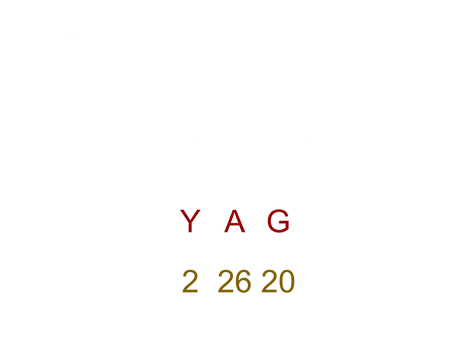# DESAIPHER

Software## BACKGROUND INFO

What is a Shift?
A shift is a move (data) one or more places to the right or left. The Letter A if it was shifted 2 then it would be the letter C.## DESAIPHER

Mohid Fawad. Kasey Doan, Niklesh Giragama

Our project is a Caesar cipher. But it is a more complex version. The shift would be a word. The letters in the word would represent a number. Instead of only using numerical values. We use the first letter in the alphabet which is 26 and the last letter would be 1. For example, Z=1, B=25, Y=2, and A=26. The shift could be a word such as BOY. The shift would then be 25, 12, and 2. If the phrase is “Desai”, D would be C, E would be Q, S would be U, A would be Z, I would be U. The final phrase would be C Q U Z U. The first letter would be shifted by 25, the second letter would be shifted by 12, and the third letter would be shifted 2. Then you would repeat it since you have gone through three letters. If the word is BOY, then you would repeat after 3 letters.

https://crypto.interactive-maths.com/caesar-shift-cipher.html

## CRACKING THE CODE

1. Find the shift word

2. Find the corresponding numbers to each of the letters in the word

3. Starting shifting each letter by their corresponding number to the word to be encrypted (repeat if your word to be encrypted is longer than the shift word)

4. You now have the encrypted word!

## DESAIPHER SERVICES## GET IN TOUCH

San Jose, California